• 高斯概率分布(Gaussian probability distributions)描述了许多噪声过程，我们应该看看它的数学公式。从一个非常简单的公式开始，考虑高斯概率分布的“钟形曲线(bell curve)”公式：无需绘制图片，我们知道公式1所...
这个模拟世界中的各种物理过程都表现出一定程度的随机性，例如，请想想噪声。高斯概率分布(Gaussian probability distributions)描述了许多噪声过程，我们应该看看它的数学公式。从一个非常简单的公式开始，考虑高斯概率分布的“钟形曲线(bell curve)”公式：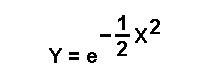无需绘制图片，我们知道公式1所描述的曲线在x值为零时具有y值，并且我们进一步知道随着x值朝向任一值，y值都变为零，负无穷大或正无穷大。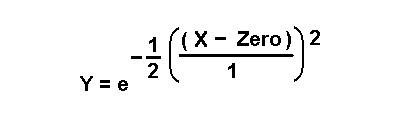接下来，依照上面的公式添加“零”和数字“ 1”，这实际上并没有改变任何内容，但是会引导我们进行下一步。下面的公式3引入了平均值和标准偏差。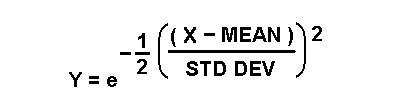本文的“平均值”是一个新的中心值，围绕它的x值更改将影响y值。现在，当x值等于平均值时，y值将变为1，其中平均值可以为零，也可以为任何非零值。“标准偏差(standard deviation)”将影响随着x值偏离均值，y值向零下降的速度有多快。标准偏差的较大值将要求x值与平均值相差很大，从而明显降低y值。 另一方面，当标准偏差较小时，x值与均值的微小偏差将使y值更快地变为零。现在，绘制几张图片，以图形方式查看均值和标准偏差如何完成各自的工作(图1)。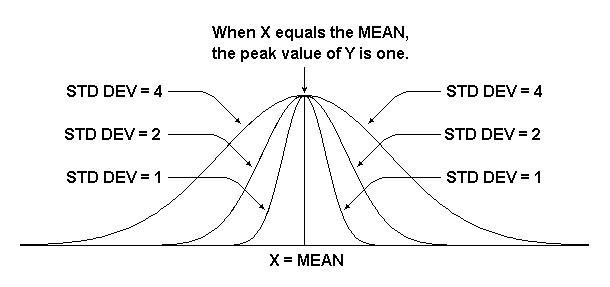图1 显示平均值和标准偏差的影响。接下来，透过标准偏差的倒数来缩放y值。请不要担心为何我们要这样做，请继续往下看。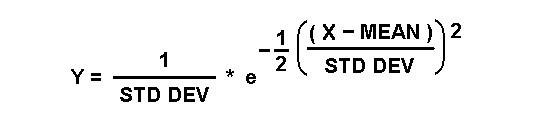如果再仔细看一下图片，会看到标准偏差的附加影响(图2)。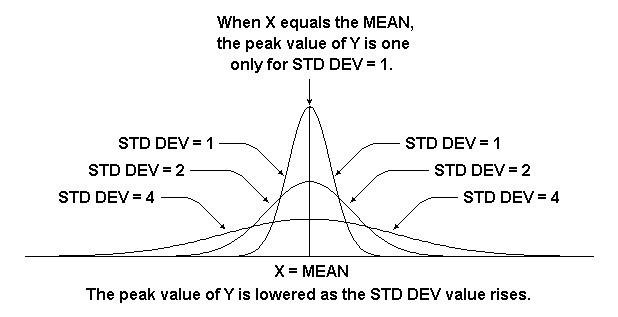图2 显示标准偏差的附加影响。接下来，输入另一个比例因子，即2 *π的平方根的倒数。再度呼吁，不用担心为什么，只要继续看下去。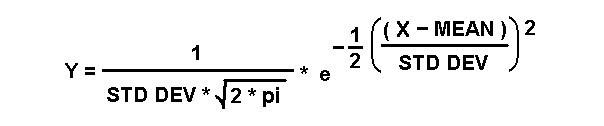现在，导入一个称为“方差(variance)”的新术语。方差只是标准偏差的平方。可以将公式重写为：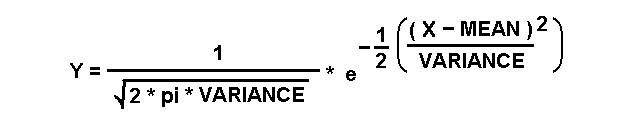至此，我们已经完成了。如图2所示，如果针对从负无穷大到正无穷大的x值范围内的y值整合这最后两个公式中的任何一个并进行积分，则曲线下的面积等于1或等于1。使用我们进行的看似任意缩放，最后两个公式中的任何一个将产生一个积分结果。无论你选择的是平均值、标准偏差还是方差，积分总是为1，这就是高斯概率分布，可以随意选择。现在，我想谈谈作为数学新手所经历的一个问题。我试着理解卡尔·弗里德里希·高斯(Carl Friedrich Gauss)等如此伟大大师的作品。然而高斯工作时，纯粹是行动上的智慧。他推断出上述公式中需要2 *π的平方根，但是高斯如何得出他的结果？我从未见过任何关于这方面的解释，也从未见过关于罗必达(l’Hospital)如何得出他寻找极限规则的任何解释，也没有见过关于帕普斯(Pappus)理论中寻找环形物体积或其他定理的任何解释。我只是被教导了这些天才努力的最终结果，以及如何应用其结果，仅此而已。不知何故，由于缺乏更深层的解释，因此我觉得被哄骗。更多技术文章，请点击了解更多
展开全文等比缩放公式
• 著名的de Moivre的Laplace极限定理从特定条件下的二项式概率质量函数证明了高斯分布概率密度函数。 De Moivre的Laplace方法非常繁琐，因为它严重依赖于许多引理和定理。 本文提出了一种可替代的，不太严格的方法，...
• 1.以下分割手部区域的代码般自博客：https://blog.csdn.net/soaringlee_fighting/article/details/72983330%% 基于高斯模型的肤色建模与似然图计算 close; clear all; clc; M = [124.2125 132.9449]' ; %肤色...
1.以下分割手部区域的代码般自博客：https://blog.csdn.net/soaringlee_fighting/article/details/72983330%% 基于高斯模型的肤色建模与似然图计算
close;
clear all;
clc;
M = [124.2125 132.9449]'  ; %肤色均值
Sigma = [75.3881  40.2587
40.2587   250.2942];  %肤色方差
Img  = imread('5.png');
figure,imshow(Img),title('原始图像');
Img2  = rgb2ycbcr(Img);%颜色空间转换
% figure,imshow(Img2(:,:,1));title('Y');
% figure,imshow(Img2(:,:,2));title('Cb');
% figure,imshow(Img2(:,:,3));title('Cr');
Img2 = double(Img2);
[m,n,dims] = size(Img);
cbcr = zeros(2,1);
for u = 1:m
for v =1:n
%x = [Img2(u,v,2),Img2(u,v,3)];
cbcr(1) = Img2(u,v,3);
cbcr(2) = Img2(u,v,2);
P(u,v) = exp(-0.5*((cbcr-M)')*(inv(Sigma))*(cbcr-M));%计算似然度
if P(u,v) < 0.20
BinImg(u,v) = 1;%生成二值图像
end
end
end
for i= 1:m
for j = 1:n
MAX = max(max(P));
Q(i,j) = (P(i,j)/MAX)*255;  %归一化
end
end
figure,imshow(Q,[]),title('肤色似然图');
figure,imshow(BinImg),title('二值化'); 2.结果图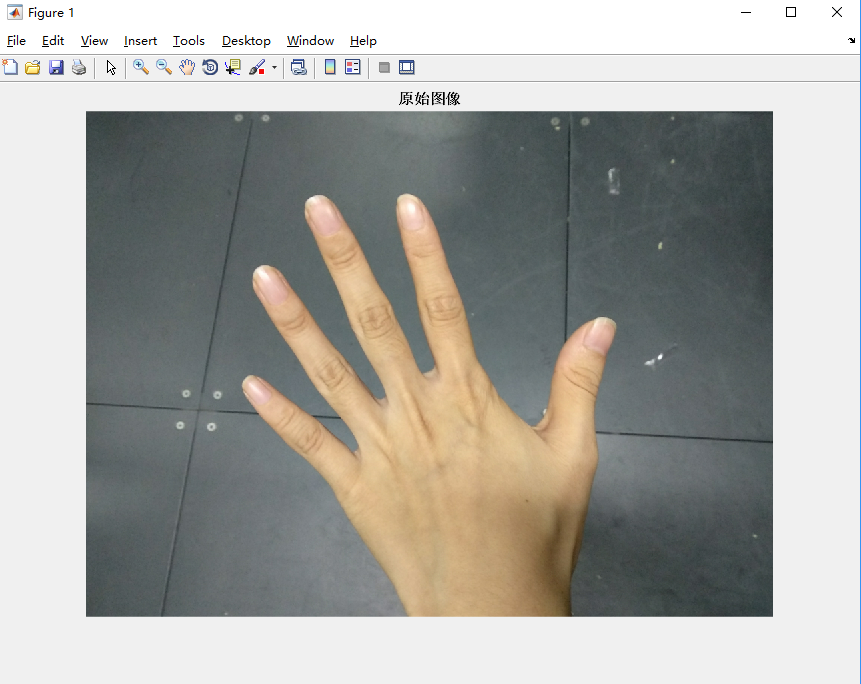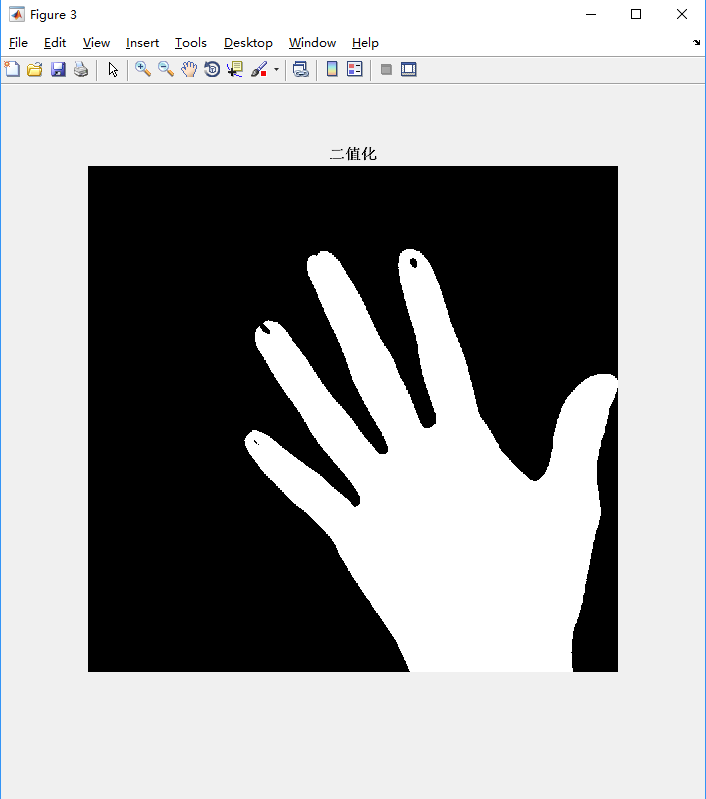3.手部分割代码理解    二维高斯型函数：    其中：x为样本像素在YCbCr空间的值x=[Cb,Cr]T，M为肤色在YCbCr空间的样本均值M=E(x)，C为肤色相似度模型的协方差矩阵C=E((x-M)(x-M)T)。    样本统计：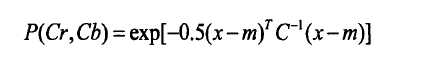为确定函数里的参数，需要采集大量的肤色样本来计算他们的统计特征。即用来得到M和C的值。                  代入高斯函数求得各个P（Cb，Cr）值，然后进行归一化处理。做法是：将Pi(Cb,Cr)/max(Pi(Cb,Cr)),用这个商作为该点的相似度值.为了查看相似度后图像，可以将 [0，1]转化为[0，255]。做法是将（Pi(Cb,Cr)/max(Pi(Cb,Cr)）*255。4.改写上面的代码绘制测试图像的二维高斯概率分布图close;
clear all;
clc;
M = [124.2125 132.9449]'  ; %肤色均值
Sigma = [75.3881  40.2587
40.2587   250.2942];  %肤色方差
Img  = imread('5.png');
figure,imshow(Img),title('原始图像');
Img2  = rgb2ycbcr(Img);%颜色空间转换
% figure,imshow(Img2(:,:,2));title('Cb');
% figure,imshow(Img2(:,:,3));title('Cr');
Img2 = double(Img2);
[m,n,dims] = size(Img);
%生成一个两行一列的零矩阵
cbcr = zeros(2,1);
zz = zeros(m,n);
for u = 1:m
for v =1:n
%x = [Img2(u,v,2),Img2(u,v,3)];
cbcr(1) = Img2(u,v,3);
cbcr(2) = Img2(u,v,2);
z = exp(-0.5*((cbcr-M)')*(inv(Sigma))*(cbcr-M));%计算似然度
%z是一个值，所以要先将所有的z存放到矩阵中，后面画图需要用到
zz(u,v)=z;
end
end
%meshgrid生成二维网格矩阵数据
%[X,Y]=meshgrid(x,y),
%x和y分别是x轴和y轴的采样的点，生成的X是采样点的横坐标，Y是纵坐标
[x,y]=meshgrid(1:1:n,1:1:m);
mesh(x,y,zz);
hold on;结果图：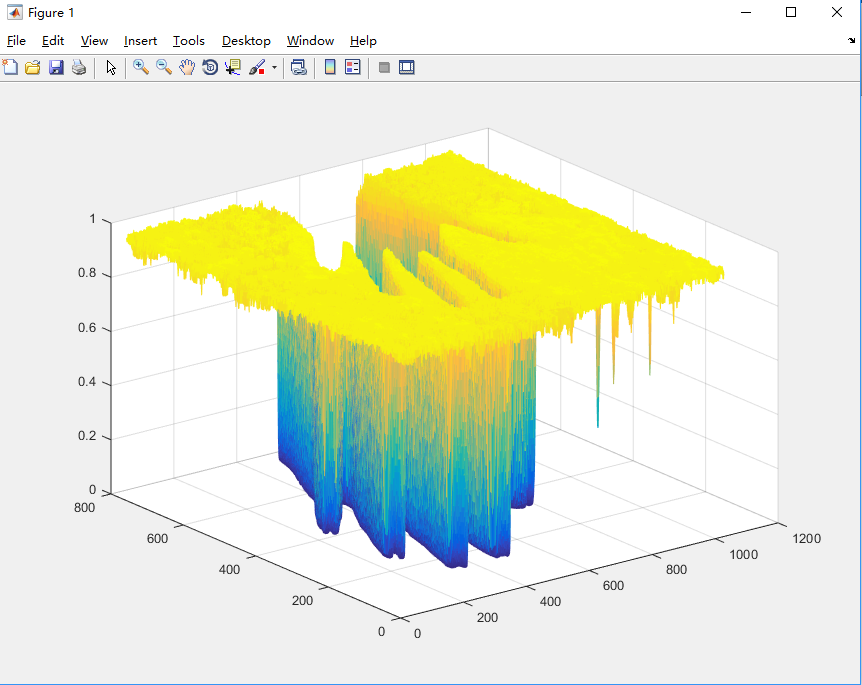5.画图说明：X和Y轴表示像素的位置，Z轴表示该像素是否属于手的概率。图像是一个m行*n列的矩阵。meshgrid用法参考博客：https://blog.csdn.net/goodshot/article/details/61208708注意：mesh(x,y,z) 这三个参数必须是矩阵。mesh用法参考博客：https://blog.csdn.net/zz501306162/article/details/54287593
展开全文•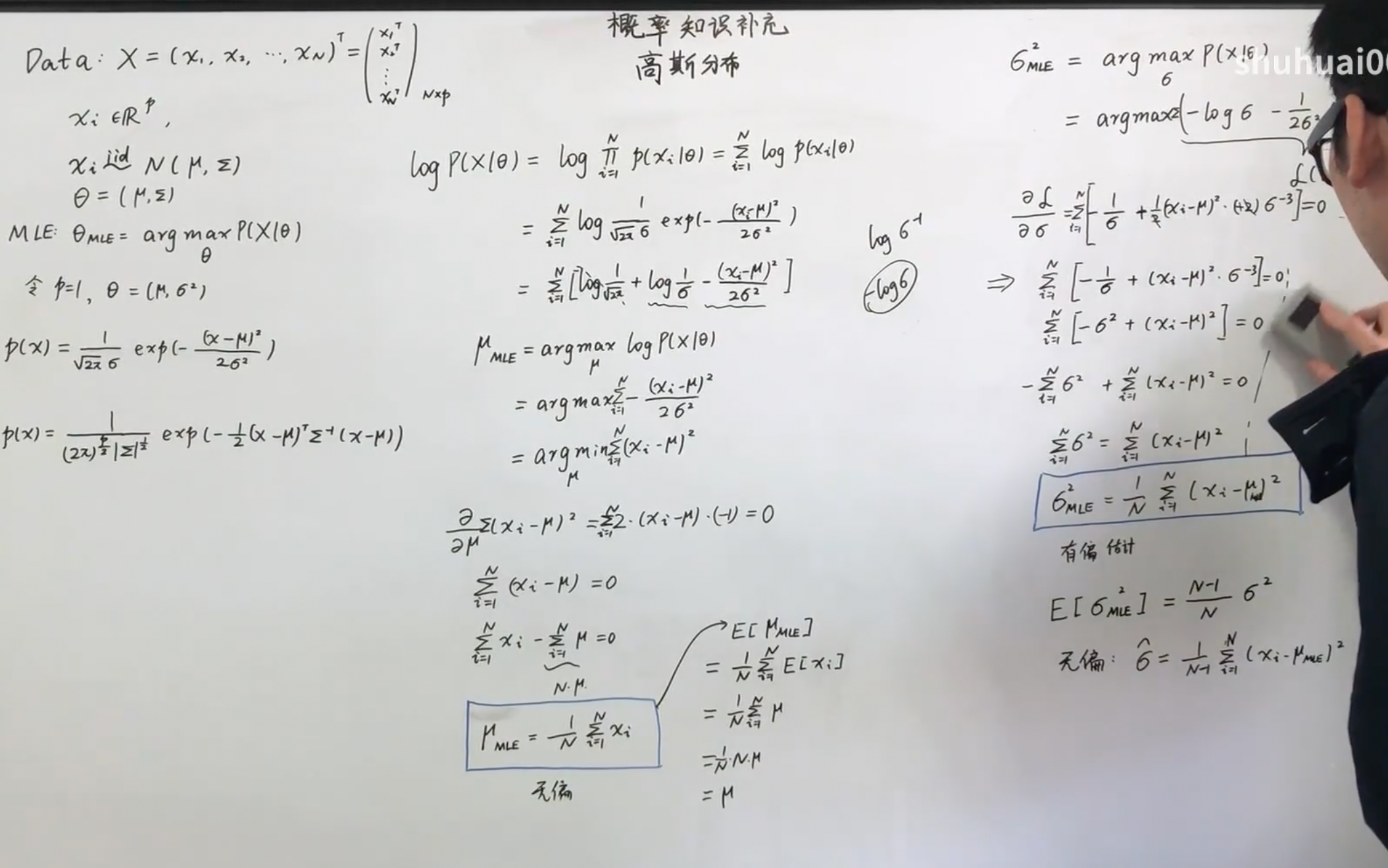展开全文• matlab开发-高斯正态分布概率密度函数。高斯正态分布是应用最广泛的分布之一。
• 上一讲对高维高斯概率分布N(μ,∑)\mathcal{N}(\mu,\sum)N(μ,∑)在定义域上积分为1进行了证明，这一讲来推导高斯分布的边缘概率和条件概率公式。推导过程与PRML一书类似，但对细节进行了展开介绍。随后介绍如何利用...
上一讲对高维高斯概率分布$\mathcal{N}(\mu,\sum)$在定义域上积分为1进行了证明，这一讲来推导高斯分布的边缘概率和条件概率公式。边缘概率可从配二次型法得到，这一讲的推导过程比PRML一书更加详细。PRML一书直接在指数部分配二次型就得到了结果，并未对其中过程进行解释，有种根据结果必为高斯分布反推过程的感觉。随后介绍如何利用高斯随机变量的线性组合公式进行推导。高维高斯分布$\mathcal{N}(\mu,\sum)$的具体公式可写为：
$\mathcal{N}(\mu,\sum)=\frac{1}{(2\pi)^{\frac{N}{2}}|\sum|^\frac{1}{2}}e^{-\frac{1}{2}(\mathbf{x}-\mu)^T\sum^{-1}(\mathbf{x}-\mu)},\ \ \ \ \ (1)$ 数据$\mathbf{x}\in\mathcal{R}^N$, $\sum$为协方差矩阵 (正定对称)。
由于$\mathbf{x}=\{\mathbf{x}_a, \mathbf{x}_b\}\sim\mathcal{N}(\mu,\sum)$, 根据概率论中的定义：边缘概率（marginal probability）${\it p}(\mathbf{x}_a)=\int_{\mathbf{x}\in\mathcal{R}^N}\it p(\mathbf{x}_a, \mathbf{x}_b)d\mathbf{x}_b$， 条件概率（conditional probability）${\it p}(\mathbf{x}_a|\mathbf{x}_b)=\frac{\it p(\mathbf{x_a}, \mathbf{x_b})}{\it p( \mathbf{x_b})}=\frac{\mathcal{N}(\mu,\sum)}{\int_{\mathbf{x}\in\mathcal{R}^N}\it p(\mathbf{x}_a, \mathbf{x}_b)d\mathbf{x}_a}$,。这两个概率的计算涉及到积分，看起来形式比较复杂。
**
1. 配二次型法
**
先假设$N=N_a+N_b$，其中$N_a$是$\mathbf{x}_a$的维度，$N_b$是$\mathbf{x}_b$的维度。同时，令$\mathbf{P}=\sum^{-1}$, 矩阵$\mathbf{P}$有一个名字叫Precision matrix。
下面是比较复杂的一步，对两个矩阵$\mathbf{P}$和$\mathbf{\sum}$根据$\mathbf{x}_a$和$\mathbf{x}_b$的维度进行分块，并利用分块矩阵求逆的公式建立关联 (这是因为高维高斯分布用到$\mathbf{\sum}^{-1}$，我们先建立前导知识)：
$\mathbf{P}=\left[\begin{array}{cc}\mathbf{P}_{aa}&\mathbf{P}_{ab} \\ \mathbf{P}_{ba}&\mathbf{P}_{bb} \end{array}\right], \ \ \ \ \sum = \left[\begin{array}{cc}\mathbf{\sum}_{aa}&\mathbf{\sum}_{ab} \\ \mathbf{\sum}_{ba}&\mathbf{\sum}_{bb} \end{array}\right],$
先从讲述矩阵Schur complement的概念开始，这个概念在求解矩阵方程中常用，其实是消元法的矩阵版本。假设：
$\sum\left[\begin{array}{c}\mathbf{x}_a\\\mathbf{x}_b\end{array}\right]=\left[\begin{array}{c}\mathbf{y}_a\\\mathbf{y}_b\end{array}\right].\ \ \ \ \ (2)$ 那么我们有：
$\mathbf{\sum}_{aa}\mathbf{x}_a+\mathbf{\sum}_{ab}\mathbf{x}_b=\mathbf{y}_a,\ \ \ \ \ (3)$$\mathbf{\sum}_{ba}\mathbf{x}_a+\mathbf{\sum}_{bb}\mathbf{x}_b=\mathbf{y}_b,\ \ \ \ \ (4)$
由(2)推出：$\mathbf{x}_b=\mathbf{\sum}_{bb}^{-1}\mathbf{y}_b - \mathbf{\sum}_{bb}^{-1}\mathbf{\sum}_{ba}\mathbf{x}_a$, 代入公式(3)可得：
$\mathbf{\sum}_{aa}\mathbf{x}_a+\mathbf{\sum}_{ab}(\mathbf{\sum}_{bb}^{-1}\mathbf{y}_b - \mathbf{\sum}_{bb}^{-1}\mathbf{\sum}_{ba}\mathbf{x}_a)=\mathbf{y}_a,\ \ \ \ \ (5)$整理可得到求解$\mathbf{x}_a$的公式为：$\mathbf{x}_a=(\mathbf{\sum}_{aa}-\mathbf{\sum}_{ab}\mathbf{\sum}_{bb}^{-1}\mathbf{\sum}_{ba})^{-1}(\mathbf{y}_a-\mathbf{\sum}_{ab}\mathbf{\sum}_{bb}^{-1}\mathbf{y}_b). \ \ \ \ \ (6)$上式中$\mathbf{M}_a =(\mathbf{\sum}_{aa}-\mathbf{\sum}_{ab}\mathbf{\sum}_{bb}^{-1}\mathbf{\sum}_{ba})^{-1}$即为矩阵$\mathbf{\sum}_{bb}$的Schur complement。注意，同理可得矩阵$\mathbf{\sum}_{aa}$的Schur complement为$\mathbf{M}_b =(\mathbf{\sum}_{bb}-\mathbf{\sum}_{ba}\mathbf{\sum}_{aa}^{-1}\mathbf{\sum}_{ab})^{-1}$。能求解$\mathbf{x}_a$和$\mathbf{x}_b$就能求出矩阵$\mathbf{\sum}$的逆矩阵：
$\mathbf{\sum}^{-1}\left[\begin{array}{c}\mathbf{y}_a\\\mathbf{y}_b\end{array}\right]=\left[\begin{array}{cc}\mathbf{M}_a&-\mathbf{M}_a\mathbf{\sum}_{ab}\mathbf{\sum}_{bb}^{-1}\\ -\mathbf{\sum}_{bb}^{-1}\mathbf{\sum}_{ba}\mathbf{M}_{a}&\mathbf{\sum}_{bb}^{-1}(\mathbf{I}+\mathbf{\sum}_{ba}\mathbf{M}_{a}\mathbf{\sum}_{ab}\mathbf{\sum}_{bb}^{-1})\end{array}\right]\left[\begin{array}{c}\mathbf{y}_a\\\mathbf{y}_b\end{array}\right]=\left[\begin{array}{c}\mathbf{x}_a\\\mathbf{x}_b\end{array}\right]\ \ \ \ (7)$
提示：逆矩阵的形式推导主要是要从$\mathbf{y}_a$和$\mathbf{y}_b$求解$\mathbf{x}_a$和$\mathbf{x}_b$。可首先把公式(6)代入公式（4）：$\mathbf{\sum}_{ba}\mathbf{M}_a(\mathbf{y}_a-\mathbf{\sum}_{ab}\mathbf{\sum}_{bb}^{-1}\mathbf{y}_b)+\mathbf{\sum}_{bb}\mathbf{x}_b=\mathbf{y}_b，\ \ \ \ (8)$整理可得：
$\mathbf{x}_b=\mathbf{\sum}_{bb}^{-1}(\mathbf{I}+\mathbf{\sum}_{ba}\mathbf{M}_{a}\mathbf{\sum}_{ab}\mathbf{\sum}_{bb}^{-1})\mathbf{y}_b-\mathbf{\sum}_{bb}^{-1}\mathbf{\sum}_{ba}\mathbf{M}_{a}\mathbf{y}_a.\ \ \ \ (9)$ $\mathbf{y}_a$和$\mathbf{y}_b$的系数即为公式(7)中的第一行。
注：矩阵$\mathbf{\sum}$的逆矩阵的另外一个形式如下：
$\mathbf{\sum}^{-1}\left[\begin{array}{c}\mathbf{y}_a\\\mathbf{y}_b\end{array}\right]=\left[\begin{array}{cc}\mathbf{\sum}_{aa}^{-1}(\mathbf{I}+\mathbf{\sum}_{ab}\mathbf{M}_{b}\mathbf{\sum}_{ba}\mathbf{\sum}_{aa}^{-1})&-\mathbf{\sum}_{aa}^{-1}\mathbf{\sum}_{ab}\mathbf{M}_{b}\\ -\mathbf{M}_b\mathbf{\sum}_{ba}\mathbf{\sum}_{aa}^{-1}&\mathbf{M}_b\end{array}\right]\left[\begin{array}{c}\mathbf{y}_a\\\mathbf{y}_b\end{array}\right]=\left[\begin{array}{c}\mathbf{x}_a\\\mathbf{x}_b\end{array}\right]\ \ \ \ (10)$
公式(7)和(10)都可以是矩阵$\mathbf{P}$的具体形式。
现在开始推导边缘概率$\it p(\mathbf{x}_a)$:
${\it p}(\mathbf{x}_a)=\int_{\mathbf{x}_b\in\mathcal{R}^N_b}\frac{1}{(2\pi)^{\frac{N}{2}}|\sum|^\frac{1}{2}}e^{-\frac{1}{2}(\mathbf{x}-\mu)^T\sum^{-1}(\mathbf{x}-\mu)}d\mathbf{x}_b \\ \\ =\frac{1}{(2\pi)^{\frac{N}{2}}|\sum|^\frac{1}{2}}\int_{\mathbf{x}_b\in\mathcal{R}^N_b}e^{-\frac{1}{2}(\left[\begin{array}{cc}(\mathbf{x}_a -\mu_a)^T&(\mathbf{x}_b-\mu_b)^T\end{array}\right])\left[\begin{array}{cc}\mathbf{P}_{aa}&\mathbf{P}_{ab} \\ \mathbf{P}_{ba}&\mathbf{P}_{bb} \end{array}\right]\left[\begin{array}{c}\mathbf{x}_a-\mathbf{\mu}_a \\ \mathbf{x}_b-\mathbf{\mu}_b\end{array}\right]}d\mathbf{x}_b \\ \\ \underset{\hat{\mathbf{x}}_a=\mathbf{x}_a-\mu_a,\hat{\mathbf{x}}_b=\mathbf{x}_b-\mu_b}{=}\frac{1}{(2\pi)^{\frac{N}{2}}|\sum|^\frac{1}{2}}\int_{\hat{\mathbf{x}}_b\in\mathcal{R}^N_b}e^{-\frac{1}{2}(\left[\begin{array}{cc}\hat{\mathbf{x}}_a^T &\hat{\mathbf{x}}_b^T\end{array}\right]\left[\begin{array}{cc}\mathbf{P}_{aa}&\mathbf{P}_{ab} \\ \mathbf{P}_{ba}&\mathbf{P}_{bb} \end{array}\right]\left[\begin{array}{c}\hat{\mathbf{x}}_a \\ \hat{\mathbf{x}}_b\end{array}\right])}d\hat{\mathbf{x}}_b \\ \\ =\frac{1}{(2\pi)^{\frac{N}{2}}|\sum|^\frac{1}{2}}\int_{\hat{\mathbf{x}}_b\in\mathcal{R}^N_b}e^{-\frac{1}{2}(\hat{\mathbf{x}}_a^T\mathbf{P}_{aa}\hat{\mathbf{x}}_a+\hat{\mathbf{x}}_a^T\mathbf{P}_{ab}\hat{\mathbf{x}}_b+\hat{\mathbf{x}}_b^T\mathbf{P}_{ba}\hat{\mathbf{x}}_a+\hat{\mathbf{x}}_b^T\mathbf{P}_{bb}\hat{\mathbf{x}}_b)}d\hat{\mathbf{x}}_b \\ \\ = \frac{1}{(2\pi)^{\frac{N}{2}}|\sum|^\frac{1}{2}}e^{-\frac{1}{2}\hat{\mathbf{x}}_a^T\mathbf{P}_{aa}\hat{\mathbf{x}}_a}\int_{\hat{\mathbf{x}}_b\in\mathcal{R}^N_b}e^{-\frac{1}{2}(2*\hat{\mathbf{x}}_b^T\mathbf{P}_{ba}\hat{\mathbf{x}}_a+\hat{\mathbf{x}}_b^T\mathbf{P}_{bb}\hat{\mathbf{x}}_b)}d\hat{\mathbf{x}}_b \\ \\ = \frac{1}{(2\pi)^{\frac{N}{2}}|\sum|^\frac{1}{2}}e^{-\frac{1}{2}\hat{\mathbf{x}}_a^T\mathbf{P}_{aa}\hat{\mathbf{x}}_a}\int_{\hat{\mathbf{x}}_b\in\mathcal{R}^N_b}e^{-\frac{1}{2}((\hat{\mathbf{x}}_b+\mathbf{P}_{bb}^{-1}\mathbf{P}_{ba}\hat{\mathbf{x}}_a)^T\mathbf{P}_{bb}(\hat{\mathbf{x}}_b+\mathbf{P}_{bb}^{-1}\mathbf{P}_{ba}\hat{\mathbf{x}}_a)-\hat{\mathbf{x}}_a^T\mathbf{P}_{ab}\mathbf{P}_{bb}^{-1}\mathbf{P}_{ba}\hat{\mathbf{x}}_a)}d\hat{\mathbf{x}}_b \\ \\ = \frac{1}{(2\pi)^{\frac{N}{2}}|\sum|^\frac{1}{2}}e^{-\frac{1}{2}\hat{\mathbf{x}}_a^T(\mathbf{P}_{aa}-\mathbf{P}_{ab}\mathbf{P}_{bb}^{-1}\mathbf{P}_{ba})\hat{\mathbf{x}}_a}\int_{\hat{\mathbf{x}}_b\in\mathcal{R}^N_b}e^{-\frac{1}{2}((\hat{\mathbf{x}}_b+\mathbf{P}_{bb}^{-1}\mathbf{P}_{ba}\hat{\mathbf{x}}_a)^T\mathbf{P}_{bb}(\hat{\mathbf{x}}_b+\mathbf{P}_{bb}^{-1}\mathbf{P}_{ba}\hat{\mathbf{x}}_a)}d\hat{\mathbf{x}}_b,$由上一讲证明高斯分布的积分结果，可得：$\int_{\hat{\mathbf{x}}_b\in\mathcal{R}^N_b}e^{-\frac{1}{2}((\hat{\mathbf{x}}_b+\mathbf{P}_{bb}^{-1}\mathbf{P}_{ba}\hat{\mathbf{x}}_a)^T\mathbf{P}_{bb}(\hat{\mathbf{x}}_b+\mathbf{P}_{bb}^{-1}\mathbf{P}_{ba}\hat{\mathbf{x}}_a)}d\hat{\mathbf{x}}_b=\frac{(2\pi)^{\frac{N_b}{2}}}{|\mathbf{P}_{bb}|^{\frac{1}{2}}}.$
同时，根据分块矩阵的行列式公式及上面的 Schur complent的推导$|\sum|=|\mathbf{\sum}_{aa}||\mathbf{\sum}_{bb}-\mathbf{\sum}_{ba}\mathbf{\sum}_{aa}^{-1}\mathbf{\sum}_{ab}|=\frac{|\mathbf{\sum}_{aa}|}{|\mathbf{M}_b|}=\frac{|\mathbf{\sum}_{aa}|}{|\mathbf{P}_{bb}|^{\frac{1}{2}}}$, 我们可得：
$\frac{1}{(2\pi)^{\frac{N}{2}}|\sum|^\frac{1}{2}}\frac{(2\pi)^{\frac{N_b}{2}}}{|\mathbf{P}_{bb}|^{\frac{1}{2}}}=\frac{1}{(2\pi)^{\frac{N_a}{2}}|\mathbf{\sum}_{aa}|^{\frac{1}{2}}}$
接下来，我们需要说明 $\mathbf{P}_{aa}-\mathbf{P}_{ab}\mathbf{P}_{bb}^{-1}\mathbf{P}_{ba}=\mathbf{\sum}_{aa}^{-1}$。此结论由公式(10)可以顺利推出，不再详细展开。因此，我们可得：
${\it p}(\mathbf{x}_a)=\int_{\mathbf{x}_b\in\mathcal{R}^N_b}\frac{1}{(2\pi)^{\frac{N}{2}}|\sum|^\frac{1}{2}}e^{-\frac{1}{2}(\mathbf{x}-\mu)^T\sum^{-1}(\mathbf{x}-\mu)}d\mathbf{x}_b=\frac{1}{(2\pi)^{\frac{N_a}{2}}|\mathbf{\sum}_{aa}|^{\frac{1}{2}}}e^{-\frac{1}{2}\hat{\mathbf{x}}_a^T\mathbf{\sum}_{aa}^{-1}\hat{\mathbf{x}}_a} \\ \\ = \frac{1}{(2\pi)^{\frac{N_a}{2}}|\mathbf{\sum}_{aa}|^{\frac{1}{2}}}e^{-\frac{1}{2}(\mathbf{x}_a-\mu_a)^T\mathbf{\sum}_{aa}^{-1}(\mathbf{x}_a-\mu_a)}.$
所以，最终的结论是 $\it p(\mathbf{x}_a)\sim\mathcal{N}(\mu_a, \mathbf{\sum}_{aa})$。
下面开始推导条件概率$\it p(\mathbf{x}_a|\mathbf{x}_b)$:
$p(\mathbf{x}_a|\mathbf{x}_b)=\frac{\frac{1}{(2\pi)^{\frac{N}{2}}|\sum|^\frac{1}{2}}e^{-\frac{1}{2}(\mathbf{x}-\mu)^T\sum^{-1}(\mathbf{x}-\mu)}}{\frac{1}{(2\pi)^{\frac{N_a}{2}}|\mathbf{\sum}_{bb}|^{\frac{1}{2}}}e^{-\frac{1}{2}(\mathbf{x}_b-\mu_b)^T\mathbf{\sum}_{bb}^{-1}(\mathbf{x}_b-\mu_b)}} = \frac{(2\pi)^{\frac{N_a}{2}}|\mathbf{\sum}_{bb}|^{\frac{1}{2}}}{(2\pi)^{\frac{N}{2}}|\sum|^\frac{1}{2}}e^{-\frac{1}{2}(\mathbf{x}-\mu)^T\sum^{-1}(\mathbf{x}-\mu)+\frac{1}{2}(\mathbf{x}_b-\mu_b)^T\mathbf{\sum}_{bb}^{-1}(\mathbf{x}_b-\mu_b)}$
先对指数部分进行变换：
$-\frac{1}{2}(\mathbf{x}-\mu)^T\mathbf{\sum}^{-1}(\mathbf{x}-\mu)+\frac{1}{2}(\mathbf{x}_b-\mu_b)^T\mathbf{\sum}_{bb}^{-1}(\mathbf{x}_b-\mu_b) \\ = -\frac{1}{2}(\left[\begin{array}{cc}(\mathbf{x}_a -\mu_a)^T&(\mathbf{x}_b-\mu_b)^T\end{array}\right])\left[\begin{array}{cc}\mathbf{P}_{aa}&\mathbf{P}_{ab} \\ \mathbf{P}_{ba}&\mathbf{P}_{bb} \end{array}\right]\left[\begin{array}{c}\mathbf{x}_a-\mathbf{\mu}_a \\ \mathbf{x}_b-\mathbf{\mu}_b\end{array}\right])+\frac{1}{2}(\mathbf{x}_b-\mu_b)^T\mathbf{\sum}_{bb}^{-1}(\mathbf{x}_b-\mu_b), \ \ \ \ (11)$由于$\mathbf{\sum}_{bb}^{-1} = \mathbf{P}_{bb}-\mathbf{P}_{ba}\mathbf{P}_{aa}^{-1}\mathbf{P}_{ab}$, 将其代入公式(11)并整理可得：
$-\frac{1}{2}((\mathbf{x}_a -\mu_a)^T\mathbf{P}_{aa}(\mathbf{x}_a -\mu_a)+(\mathbf{x}_a -\mu_a)^T\mathbf{P}_{ab}(\mathbf{x}_b -\mu_b) +(\mathbf{x}_b -\mu_b)^T\mathbf{P}_{ba}(\mathbf{x}_a -\mu_a) \\ +(\mathbf{x}_b -\mu_b)^T\mathbf{P}_{bb}(\mathbf{x}_b -\mu_b) - (\mathbf{x}_b -\mu_b)^T(\mathbf{P}_{bb}-\mathbf{P}_{ba}\mathbf{P}_{aa}^{-1}\mathbf{P}_{ab})(\mathbf{x}_b -\mu_b)) \\ = -\frac{1}{2}((\mathbf{x}_a -\mu_a)^T\mathbf{P}_{aa}(\mathbf{x}_a -\mu_a)+2*(\mathbf{x}_a -\mu_a)^T\mathbf{P}_{ab}(\mathbf{x}_b -\mu_b) + (\mathbf{x}_b -\mu_b)^T\mathbf{P}_{ba}\mathbf{P}_{aa}^{-1}\mathbf{P}_{ab}(\mathbf{x}_b -\mu_b)) \\ = -\frac{1}{2}((\mathbf{x}_a -\mu_a+ \mathbf{P}_{aa}^{-1}\mathbf{P}_{ab}(\mathbf{x}_b -\mu_b))^T\mathbf{P}_{aa}(\mathbf{x}_a -\mu_a+\mathbf{P}_{aa}^{-1}\mathbf{P}_{ab}(\mathbf{x}_b -\mu_b))),\ \ \ \ (12)$注意：在推导条件概率时$\mathbf{x}_b$需要当成已知量。因为只有已知$\mathbf{x}_b$，才可确定条件概率。
观察上式，只要$\mathbf{P}_{aa}=(\mathbf{\sum}_{aa}-\mathbf{\sum}_{ab}\mathbf{\sum}_{bb}^{-1}\mathbf{\sum}_{ba})^{-1}$和$\frac{|\mathbf{\sum}_{bb}|^{\frac{1}{2}}}{|\sum|^\frac{1}{2}}$配合起来，即：$\frac{|\mathbf{\sum}_{bb}|^{\frac{1}{2}}}{|\sum|^\frac{1}{2}}=\frac{1}{|\mathbf{\sum}_{aa}-\mathbf{\sum}_{ab}\mathbf{\sum}_{bb}^{-1}\mathbf{\sum}_{ba}|^{\frac{1}{2}}}$, 整个式子就可以成为一个积分为1的概率分布（见高斯分布数学性质即推导（一））。这部分的推导比较简单，由分块矩阵的行列式值公式$|\sum|=|\mathbf{\sum}_{bb}||\mathbf{\sum}_{aa}-\mathbf{\sum}_{ab}\mathbf{\sum}_{bb}^{-1}\mathbf{\sum}_{ba}|$即可得。因此，条件概率$p(\mathbf{x}_a|\mathbf{x}_b)$的协方差矩阵就是$(\mathbf{\sum}_{aa}-\mathbf{\sum}_{ab}\mathbf{\sum}_{bb}^{-1}\mathbf{\sum}_{ba})$。均值比较简单，从公式(12)立即可以得出均值为:$\mu_a-\mathbf{P}_{aa}^{-1}\mathbf{P}_{ab}(\mathbf{x}_b-\mu_b)=\mu_a-\mathbf{M}_a^{-1}*(-\mathbf{M}_a\mathbf{\sum}_{ab}\mathbf{\sum}_{bb}^{-1})(\mathbf{x}_b-\mu_b)=\mu_a+\mathbf{\sum}_{ab}\mathbf{\sum}_{bb}^{-1}(\mathbf{x}_b-\mu_b)$。
所以，可得出结论： $\it p(\mathbf{x}_a|\mathbf{x}_b)\sim\mathcal{N}(\mu_a+\mathbf{\sum}_{ab}\mathbf{\sum}_{bb}^{-1}(\mathbf{x}_b-\mu_b), \mathbf{\sum}_{aa}-\mathbf{\sum}_{ab}\mathbf{\sum}_{bb}^{-1}\mathbf{\sum}_{ba})$。


展开全文•机器学习
• （百度说的概率函数一般指概率分布函数，但课件里边提到概率函数时是如下意思↓） 离散型随机变量的分布的表现形式 注：截图来自同济大学概率论与数理统计课件 2.概率密度函数： 在数学中，连续型随机变量的...
• 一、一元高斯分布概率密度 均值为0，方差为1的标准一元正态分布概率密度函数为：在均值为 ，方差为 的情况下，需要标准化一下: ，标准化之后方差变为1，标准化的意义在于将数据点 到均值 的距离转化为数据点 到...
• 这个系列打算跟大家讲讲一些概率分布，一些大家可能知道但又不那么了解的概率分布，这里我们不会像教科书那么样侧重具体的内容，而是希望大家从理解的角度去重新认识这些概率分布，因为深入的理解才会在你想要解决某...
• 已知 D 维向量 x，其高斯概率分布为：N(x|μ,Σ)
• 条件高斯分布 边缘高斯分布 高斯变量的贝叶斯定理 高斯分布的最大似然估计 顺序估计 高斯分布的贝叶斯推断 ...
• 高斯分布： $f(x) = \frac{1}{\sqrt{2\pi }\sigma }exp(-\frac{(x-\mu)^{2}}{2\sigma^{2}})$ ...一个高斯分布只需线性变换即可化为标准高斯分布，所以只需推导标准高斯分布概率密度的积分。由...
• 高斯分布区间概率计算在Excel的应用，孔建新，，高斯分布数学模型解决了一个既能描述正态分布又能描述捧场分布的问题。从而引出了左期望差和右期望差的新概念。然而在计算机的
• 一，多元高斯分布 考虑⾼斯分布的⼏何形式，⾼斯对于 x\boldsymbol{x}x 的依赖是通过下⾯形式的⼆次型公式(2.30)： Δ2=(x−μ)TΣ−1(x−μ) \Delta^{2} = (\boldsymbol{x} - \boldsymbol{\mu})^{T} \boldsymbol{\...机器学习
• 文章目录多元高斯分布直观理解求边缘概率，条件概率求联合概率 多元高斯分布直观理解 求边缘概率，条件概率 求联合概率
• MATLAB 高斯（正太）分布MATLAB 高斯（正太）分布 MATLAB 高斯（正太）分布 英文：Normal Probability Distribution Function y = normpdf(x) y = normpdf(x,mu) y = normpdf(x,mu,sigma) 输入参数： x - 用于计算 ...matlab
• 今天小编就为大家分享一篇python高斯分布概率密度函数的使用详解，具有很好的参考价值，希望对大家有所帮助。一起跟随小编过来看看吧
• 高斯分布是一类非常重要的概率分布，在概率统计，机器学习中经常用到。 一维高斯分布 一维高斯分布的概率密度函数（pdf）形式为： 红色的曲线是标准的正态分布，即均值为0，方差为1的正态分布。 我们可以...
• 机器学习——概率分类(三)高斯概率密度与混合高斯模型 在之前的文章机器学习——概率分类(一)朴素贝叶斯模型一文中，我们主要介绍了机器学习中的概率分类问题。我们提出了简单的朴素贝叶斯模型来进行概率密度的估计...
• 正态分布(Normal distribution)又名高斯分布(Gaussian distribution)，是一个在数学、物理及工程等领域都非常重要的概率分布，在统计学的许多方面有着重大的影响力。 概率密度函数 若随机变量X服从一个数学期望为μ...
• I . 高斯混合模型方法 ( GMM ) II . 硬聚类 与 软聚类 III . GMM 聚类结果概率的作用 IV . 高斯混合分布 V . 概率密度函数 VI . 高斯分布 曲线 ( 仅做参考 ) VII . 高斯混合模型 参数简介数据挖掘
• 一、推导过程: 二、结果： 边缘分布 x1,x2 各自依然服从 μi，写反差矩阵 Σii 的多元高斯分布；...条件概率分布 给定 xj 求 xi 的分布： μi|j=μi+ΣijΣ−1jj(xj−μj) Σi|j=Σjj−ΣTijΣ−1iiΣij ...机器学习
• Author: PanDate:2020/7/15首先，我们通过随机向量的介绍来引出多元高斯分布，通过多元高斯分布，我们将介绍概率图的条件独立性假设。先快速过一遍随机向量：1.随机向量和其基本性质假设随机向量,那么假设它有个累积...
• 由于需要，网上找了函数表写了个求概率函数，对于标准正态分布只需两个参数，即所求概率区间上下限，上下限不分前后；对于一般正态分布需四个参数，即所求概率区间上下限和正态分布的均值及方差，上下限不分前后；...C#
• 概率中，最有名的两类定理，一个叫大数定理，另一个叫中心极限定理，中心极限就是正态分布，看看用词，叫“中心”。 高斯分布这么牛，为什么？怎么发现的，又有那些独特性质，来围观一下。 一 发现高斯分布 事实......